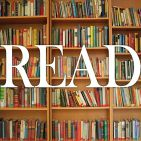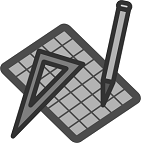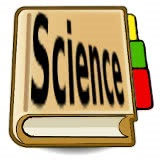## Search form## Each theme based unit covers all the main subject areas and includes lesson objective, common core standards, printable student handouts and key learning points.

Plants (Science - GR 1) Lesson Objective: To learn about the life cycle of a plant.

Plants (Writing - GR 1) Lesson Objective: To write an informational piece about the life cycle of a plant

Plants (Math - GR 1) Lesson Objective: To use addition to solve word problems

Plants (Math - GR 2)    Lesson Objective: To estimate and then measure the height of different plants

Plants (Science - GR 2) Lesson Objective: To learn about what a plant needs to grow

Plants (Writing - GR 2) Lesson Objective: To write a story from the point of view of a redwood treeHarvest - Science (GR K) Lesson Objective: To learn about different locations to grow vegetables

Harvest - Math (GR K) Lesson Objective: To compare groups of objects to identify greater than, less than and equal to

Harvest - Writing (GR K) Lesson Objective: To write an informational piece about what a harvest is.

Harvest - Reading (GR 1) Lesson Objective: To listen to the book, Strega Nona’s Harvest, and then answer questions about the book

Harvest - Science (GR 1) Lesson Objective: To learn about how to plant vegetables in a garden

Harvest - Math (GR 1) Lesson Objective: To compare the length of two objects by using a third

Harvest - Writing (GR 1) Lesson Objective: To write an opinion piece about which vegetable the student would most like to grow and why

Harvest - Writing (GR 2)  Lesson Objective: To write a story about what it would be like at a harvest party

Harvest - Social Studies (GR 2) Lesson Objective: To learn about and compare harvesting in pre-industrial times to harvesting now and how it has changed peoples’ lives

Harvest - Math (GR 2) Lesson Objective: To determine if a group of objects has an odd or even number

Harvest - Reading (GR 3)   Lesson Objective: To read an excerpt from Little House in the Big Woods and use it as a starting point for a discussion about harvesting food.

Harvest - Writing (GR 3)  Lesson Objective: To write an opinion on if harvesting was easier 100 years ago or now

Harvest - Social Studies (GR 3) Lesson Objective: To learn about and compare harvesting in pre-industrial times to harvesting now and how it has changed peoples’ lives.

Harvest - Math (GR 3) Lesson Objective: To use multiplication and division to solve equations.Baseball - Writing (GR 1) Lesson Objective: To write an opinion piece about baseball.

Baseball - Reading (GR 1) Lesson Objective: To listen to the book, The Berenstain Bears Go Out For the Team, and then answer questions about the book

Baseball - Social Studies (GR 1) Lesson Objective: To learn about baseball and answer questions

Baseball - Math (GR 1) Lesson Objective: To tell time to the hour and half-hour.

Baseball - Writing (GR 2)  Lesson Objective: To write a story about what it would be like to be the first person to do something importantBaseball - Writing (GR 3) Lesson Objective: To write a story from the point of view of a baseball

Baseball - Social Studies (GR 2) Lesson Objective: To learn about Jackie Robinson. Ask and answer such questions as who, what, where, when, why, and how to demonstrate understanding of key details in a text.

Baseball - Math (Gr 2) Lesson Objective: To practice math facts using addition doubles

Baseball - Writing (GR 3)  Lesson Objective: To write a story from the point of view of a baseball

Baseball - Social Studies (GR 3) Lesson Objective: To learn about the history of baseball in America and answer questions about what has been learned

Baseball - Math (GR 3) Lesson Objective: To use multiplication and division to solve equationsAnimal Homes - Reading (GR 1)  Lesson Objective: To listen to the book, No Place Like Home, and then answer questions about the book

Animal Homes - Science (GR 1)  Lesson Objective: To learn about two different animal homes. What is the difference between a beaver and a prairie dog?

Animal Homes - Math (GR 1) Lesson Objective: To order objects by length. Look at the pictures of the animal homes. Use the picture of the book to help you answer the questions.

Animal Homes - Writing (GR 1) Lesson Objective: To write a story about what it would be like to be an animal building a home

Animal Homes - Writing (GR 3) Lesson Objective: To write an imagined narrative about what type of home you would have if you were an animal

Animal Homes - Science (GR 3)  Lesson Objective: To learn about different types of animal homes

Animal Homes - Reading (GR 3)  Lesson Objective: To read a poem about animal homes and answer questions while referring to different stanzas of the poem

Animal Homes - Math (GR 3) Lesson Objective: To determine the perimeter of different shaped animal homes given the side lengthsInsects - Writing (GR 1) Lesson Objective: To write a story about being a helpful insect.

Insects - Math (GR 1) Lesson Objective: To use addition and subtraction to solve word problems

Insects - Science (GR 1) Lesson Objective: To learn about what makes an insect an insect.

Insects - Writing (Gr 2) Lesson Objective: To write a narrative pretending to be an insect and describing what your body looks like

Insects - Math (Gr 2) Lesson Objective: To practice math facts using addition and subtraction

Insects - Science (Gr 2) Lesson Objective: To learn about what makes an insect an insect

Insects - Math (GR 3) Lesson Objective: To use multiplication and division to solve equations

Insects - Reading (GR 3) Lesson Objective: To read a chapter from James and the Giant Peach and then answer questions relating to what was read.

Insects - Writing (GR 3) Lesson Objective: To write a story about what the world would be like without insects

Insects - Science (GR 3) There are over a million different types of insects in the world. There are insects that crawl and insects that fly.Hockey - Reading (GR 1) Lesson Objective: To listen to Dino-Hockey and then answer questions about the book.

Hockey - Writing (GR 1)  Lesson Objective: To write an opinion piece about hockey.

Hockey - Writing (GR 2) Lesson Objective: To write a story about what it would be like to be one of the first people to play hockey

Hockey - Writing (GR 3) Lesson Objective: To write a story in the perspective of a hockey puck

Hockey - Math (GR 1) Lesson Objective: To tell time to the hour and half-hour.

Hockey - Math (GR 2) Lesson Objective: To solve word problems involving time with the sport of hockey.

Hockey - Math (GR 3) Lesson Objective: To solve hockey word problems involving time

Hockey - Social Studies (GR 1)  Lesson Objective: Ask and answer questions about key details in a text.

Hockey - Social Studies (GR 2)  Lesson Objective: To learn about the game of hockey and how it started

Hockey - Social Studies (GR 3)  Lesson Objective: To learn about the history of hockey and answer questions about what has been learnedHibernation - Writing (GR K) Lesson Objective: To write an informational piece about how frogs hibernate

Hibernation - Math (GR K) Lesson Objective: To identify shapes

Hibernation - Science (GR K) Lesson Objective: To learn about how frogs hibernate

Hibernation - Writing (GR 1) - Hibernation Lesson Plan Objective: To write an opinion piece about what food they would eat to get ready to hibernate

Hibernation - Math (GR 1) - Hibernation Lesson Plan Objective: To use addition and subtraction to solve word problems regarding hibernation.

Hibernation - Science (GR 1) - Hibernation Lesson Plan Objective: To learn about why and how animals hibernate.

Hibernation - Reading (GR 1) - Hibernation Lesson Plan Objective: To read the poem, “Winter Sleep,” and then answer questions about the poem.

Hibernation - Math (Gr 2) Lesson Objective: To use addition and subtraction to solve word problems

Hibernation - Math (Gr 3) - Lesson Objective: To use addition and subtraction to solve word problems involving time in regards to hibernation

Hibernation - Reading (Gr 3) - Lesson Objective: To read a myth about why bears hibernate and discuss the message conveyed in the myth.

Hibernation - Writing (Gr 2) Lesson Objective: To read the book, Sleep, Big Bear, Sleep and answer questions about the story.

Hibernation - Writing (Gr 3) - Lesson Objective: To write an imagined narrative about hibernation

Hibernation - Science (Gr 2) Lesson Objective: To learn about animals that hibernate and then answer questions about them.

Hibernation - Science (Gr 3) - Lesson Objective: To learn why animals hibernate and then answer questions related to that informationMigration - Reading (GR K) Lesson Objective: To read the book, Home at Last: A Song of Migration, and then answer questions about the book

Migration - Writing (GR K) Lesson Objective: To write an informational piece about migration

Migration - Math (GR K) Lesson Objective: To compare two groups of objects

Migration - Science (GR K) Lesson Objective: To learn about why animals migrate.

Migration - Reading (Gr 1) Lesson Objective: To listen to Boundless Sky and then answer questions about the book

Migration - Writing (Gr 1) Lesson Objective: To write a text sharing what was learned about migration

Migration - Math (Gr 1)  Lesson Objective: To compare two digit numbers based on tens and one

Migration - Science (Gr 1)   Lesson Objective: To learn about bird migration

Migration - Writing (Gr 2) Lesson Objective: To write a story explaining if you would like to migrate and why

Migration - Math (Gr 2)  Lesson Objective: To determine if a group of objects has an odd or even number

Migration - Science (Gr 2)  Lesson Objective: To learn about why animals migrate and then answer questions about them

Migration - Writing (Gr 3)  Lesson Objective: To write an opinion indicating whether it is better for animals that migrate to live in groups or not

Migration - Math (Gr 3)   Lesson Objective: To use multiplication and division to solve and write word problems

Migration - Science (Gr 3)   Lesson Objective: To learn what migration is and why and how animals migrateSnowflakes - Writing - (Gr 3) Lesson Objective: To write an imagined narrative about being a snowflake

Snowflakes - Math - (Gr 3) Lesson Objective: To use multiplication and division to solve word problems

Snowflakes - Science - (Gr 3) Lesson Objective: To learn what snowflakes are and how they are formed

Snowflakes - Writing (Gr2)  Lesson Objective: To write an informational piece explaining what was learned about snow

Snowflakes - Math (Gr2)  Lesson Objective: To answer questions based on a bar graph

Snowflakes - Reading (Gr1) Lesson Objective: To listen to Snow Globe Family and then answer questions about the book

Snowflakes - Writing (Gr1)  Lesson Objective: To write a story about what it would be like to be a snowflake

Snowflakes - Science (Gr1) Lesson Objective: To learn about how a snowflake is formed.

Snowflakes - Math (Gr1) Lesson Objective: To use addition and subtraction to solve word problems

Snowflakes - Writing (Gr K) Lesson Objective: Teach students to write an informational piece about what a snowflake is and then draw a picture with this lesson and printable student handout

Snowflakes - Science (Gr K) Lesson Objective: Teach students about snowflakes is with this ready-to-use lesson and printable student handout.

Snowflakes - Math (Gr K) Lesson Objective:: To compare the height of two objects.Football - Reading (Gr K)  Lesson Objective: To read the book, Don’t Throw it to Mo!, and then answer questions about the book.

Football - Writing (GR K)  Lesson Objective: To write an informational piece about what football is

Football - Social Studies (Gr K)  Lesson Objective: To learn about football and answer questions.

Football - Math (Gr K)  Lesson Objective: To compare the length of two objects

Football - Writing (GR 1)  Ready to use Emergency Sub Lesson & handout!  Lesson Objective: To write an opinion piece about football.

Football - Social Studies (Gr1)  Step-by-step Emergency Sub Lesson & handout!  Lesson Objective: To learn about football and answer questions.

Football - Math (Gr1) Are you ready to play football?  Ready to use Emergency Sub Lesson & Student handout on how to use add and subtract within 10.

Football - Reading (Gr1)  Ready to use Emergency Sub Lesson & handout!  Lesson Objective: To listen to "Miss Nelson Has a Field Day" and then answer questions about the book

Football - Writing (GR 2) Lesson Objective: To write a story about what it would be like to be the football in a game

Football - Writing (Gr 3) - Lesson Objective: To write the rest of the story based on the chapter read from Touchdown Kid.

Football - Math (Gr2) Lesson Objective: To practice math facts using addition and subtraction

Football - Math (Gr 3) - Who's ready for football? Use multiplication and division to solve equations!

Football - Social Studies (Gr2) Lesson Objective: To learn about the game of football including the rules and scoring

Football - Social Studies (Gr 3) - Lesson Objective: To learn about the history of football in America and answer questions about what has been learned.Stars - Reading (GR K) Lesson Objective: To read the book, How to Catch a Star, and then answer questions about the book.

Stars - Writing (GR K) Lesson Objective: To write an informational piece about what a star is. CCSS.ELA-LITERACY.W.K.2

Stars - Science (GR K) Lesson Objective: To learn about what a star is. CCSS.ELA-LITERACY.RI.K.1

Stars - Math (GR K) Lesson Objective: Solve addition and subtraction word problems, and add and subtract within 10. CCSS.MATH.CONTENT.K.OA.A.2

Stars - Reading (GR 1) Lesson Objective: To listen to chapter 1 in Stink Moody in Master of Disaster and then answer questions about the book

Stars - Writing (GR 1) Lesson Objective: To write a narrative about star gazing. Write about a time that you looked at stars.

Stars - Science (GR 1) Lesson Objective: To learn facts about stars. Read about stars. Then, fill in the chart.

Stars - Math (GR 1) Lesson Objective: To use addition to solve problems

Stars - Writing (GR 2)  Lesson Objective: To write a narrative on a star’s name including details on why that name was chosen

Stars - Science (GR 2)  Lesson Objective: To learn about stars and then answer questions about them

Stars - Math (GR 2) Lesson Objective: To use addition and subtraction to solve word problems

Stars - Writing (GR 3) Lesson Objective: To write a myth about an imagined constellation

Stars - Science (GR 3) - Lesson Objective: learn about how a star is formed and then answer questions related to that information

Stars - Math (GR 3)  - Lesson Objective: To use multiplication and division to solve word problems.

Stars - Reading (GR 3) - Objective: a myth about a constellation and discuss the message conveyed in the myth.Life-cycle - Writing (Gr K) Lesson Objective: To write an informational piece about the cycle of a butterfly.

Life-cycle - Science (Gr K) Lesson Objective: To learn about the life cycle of a butterfly

Life-cycle - Math (Gr K)  Lesson Objective: To count to answer the question ‘how many?’

Life-cycle - Writing (GR 1) Lesson Objective: To write an informational piece about a life cycle

Life-cycle - Science (GR1) Lesson Objective: To learn about the life cycle of a chick and the life cycle of a dog and compare the life cycles.

Life-cycle - Math (GR 1)  Lesson Objective: To use addition and subtraction to solve word problems

Life Cycle - Reading (GR 2) - Lesson Objective: To read the story, The Frog Prince, and then answer questions about the story.

Life Cycle - Writing (GR 2) - Lesson Objective: To write an opinion about how they would react to meeting the Frog Prince.

Life Cycle - Science (GR 2) - Lesson Objective: To learn about where frogs life, the life cycle of frogs and then answer questions about the life cycle

Life Cycle - Math (GR 2) - Lesson Objective: To use addition and subtraction to solve word problems

Life Cycle - Math (GR 3) - Lesson Objective: To use multiplication and division to solve word problems

Life Cycle - Science (GR 3) - Lesson Objective: To learn about the life cycle of trumpeter swans and then answer questions about the life cycle

Life Cycle - Reading (GR 3) -  Lesson Objective: To read an excerpt from Trumpet of the Swans and discuss how it relates to the life cycle of swans.

Life Cycle - Writing (GR3) - Lesson Objective: To write the rest of the story based on the chapter read from The Trumpet of the Swan